# Newtons second law Objectives Explain the effect of

• Slides: 48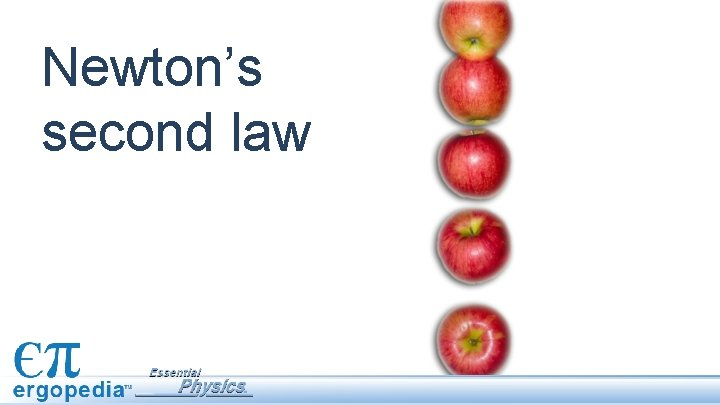Newton’s second law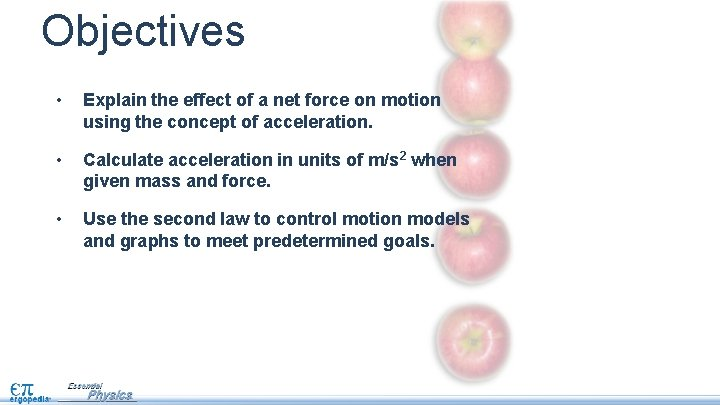Objectives • Explain the effect of a net force on motion using the concept of acceleration. • Calculate acceleration in units of m/s 2 when given mass and force. • Use the second law to control motion models and graphs to meet predetermined goals.Assessment 1. A net force of 10 N acts on a cart on a straight track. Label each statement below as true, possibly true, or false. a. The cart moves with constant velocity. b. The cart moves with constant acceleration. c. The cart speeds up. d. The cart slows down.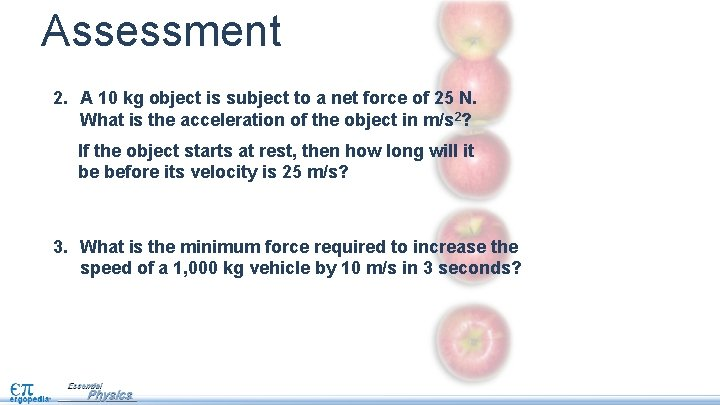Assessment 2. A 10 kg object is subject to a net force of 25 N. What is the acceleration of the object in m/s 2? If the object starts at rest, then how long will it be before its velocity is 25 m/s? 3. What is the minimum force required to increase the speed of a 1, 000 kg vehicle by 10 m/s in 3 seconds?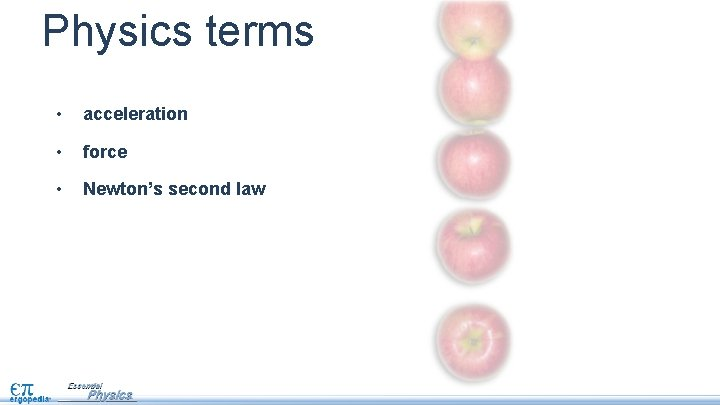Physics terms • acceleration • force • Newton’s second law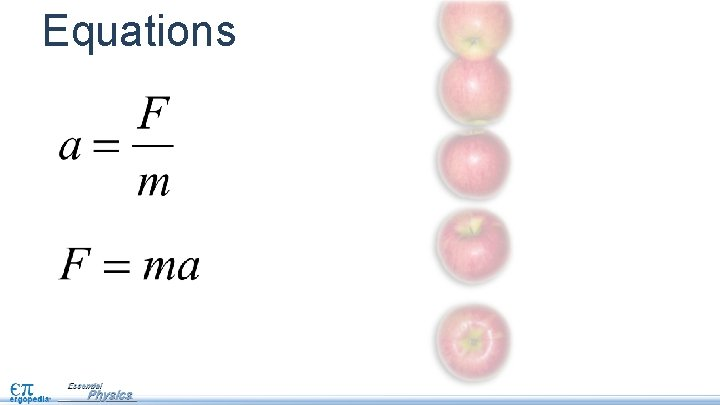Equations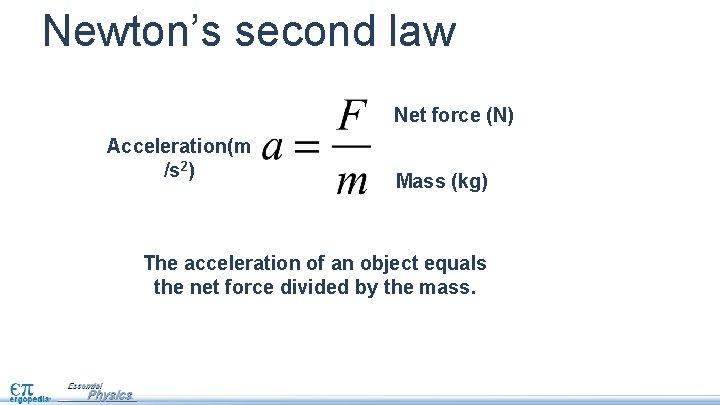Newton’s second law Net force (N) Acceleration(m /s 2) Mass (kg) The acceleration of an object equals the net force divided by the mass.Newton’s second law Net force (N) Acceleration(m /s 2) Mass (kg) Velocity must change if a net force acts on an object. How does the second law show that this statement is true?The meaning of the second law Net force (N) Acceleration(m /s 2) Mass (kg) Velocity must change if a net force acts on an object. According to the second law, a net force on an object causes it to accelerate. If an object accelerates, its velocity must change.The meaning of the second law Net force (N) Acceleration(m /s 2) Mass (kg) The net force is zero on an object with constant velocity. How does the second law show that this statement is true?The meaning of the second law Net force (N) Acceleration(m /s 2) Mass (kg) The net force is zero on an object with constant velocity. If velocity stays constant, then the acceleration is zero—so the net force must also be zero.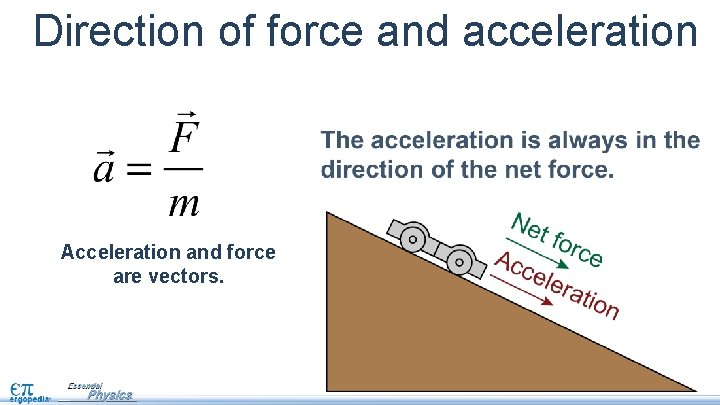Direction of force and acceleration Acceleration and force are vectors.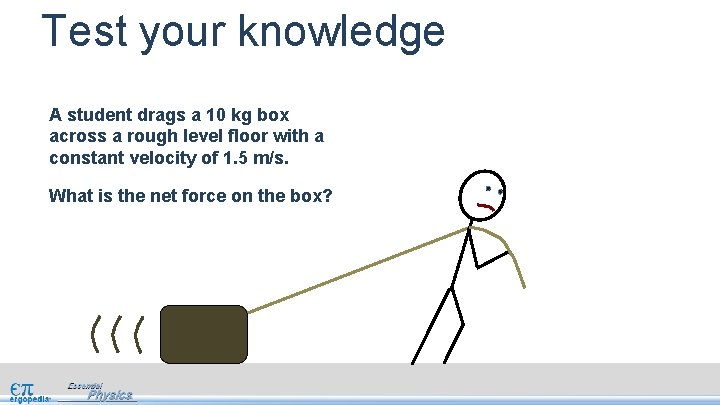Test your knowledge A student drags a 10 kg box across a rough level floor with a constant velocity of 1. 5 m/s. What is the net force on the box?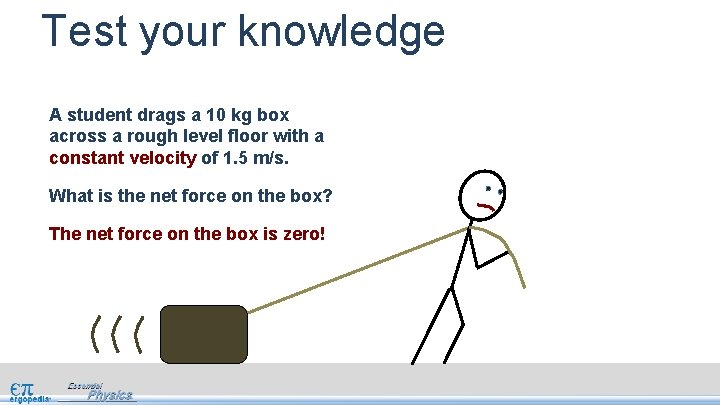Test your knowledge A student drags a 10 kg box across a rough level floor with a constant velocity of 1. 5 m/s. What is the net force on the box? The net force on the box is zero!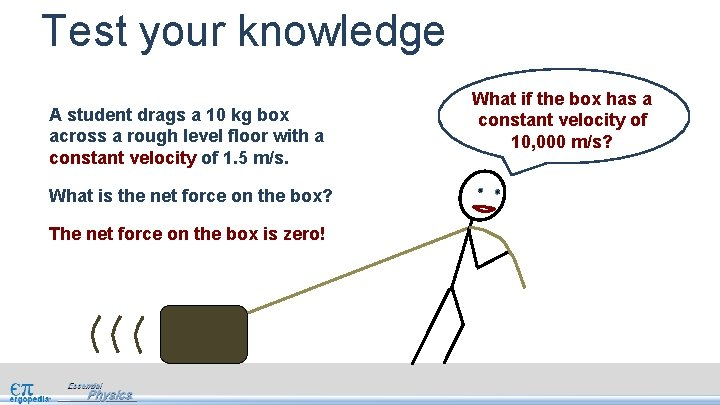Test your knowledge A student drags a 10 kg box across a rough level floor with a constant velocity of 1. 5 m/s. What is the net force on the box? The net force on the box is zero! What if the box has a constant velocity of 10, 000 m/s?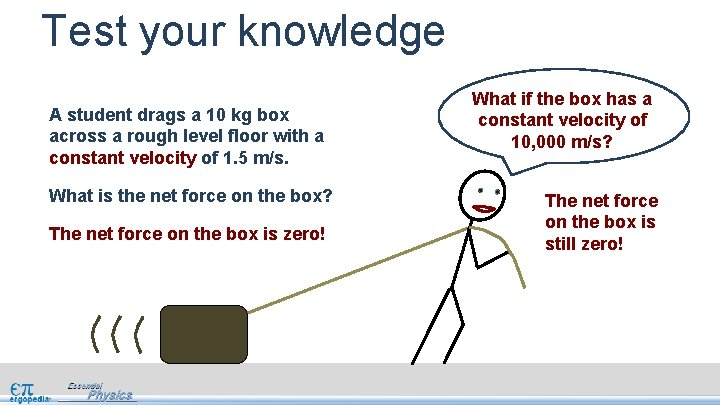Test your knowledge A student drags a 10 kg box across a rough level floor with a constant velocity of 1. 5 m/s. What is the net force on the box? The net force on the box is zero! What if the box has a constant velocity of 10, 000 m/s? The net force on the box is still zero!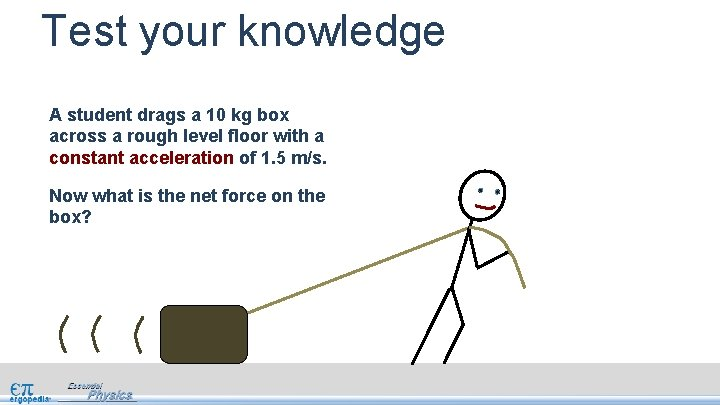Test your knowledge A student drags a 10 kg box across a rough level floor with a constant acceleration of 1. 5 m/s. Now what is the net force on the box?Test your knowledge A student drags a 10 kg box across a rough level floor with a constant acceleration of 1. 5 m/s. Now what is the net force on the box? Easy! Fnet = ma = 15 N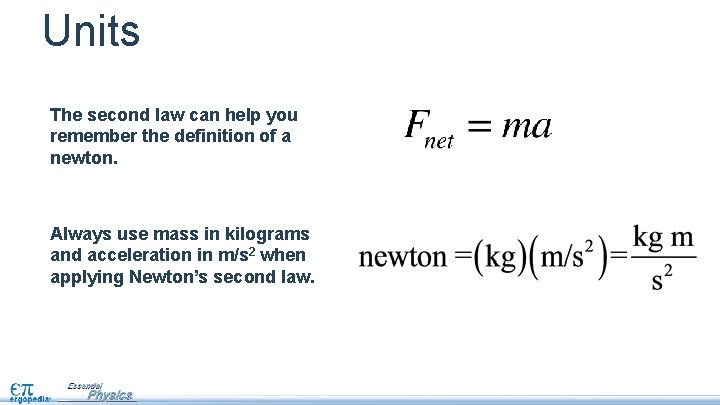Units The second law can help you remember the definition of a newton. Always use mass in kilograms and acceleration in m/s 2 when applying Newton’s second law.Exploring the ideas Click this interactive calculator on page 141.Engaging with the concepts You can solve force, mass, or acceleration. Use the calculator to answer sample questions. Click the [Run] button to see the hand apply a force to push the ball.Engaging with the concepts A net force of 500 N acts on a 100 kg cart. What is the acceleration? If you double the mass of the cart, what is the acceleration? What if the force is doubled instead? 500 100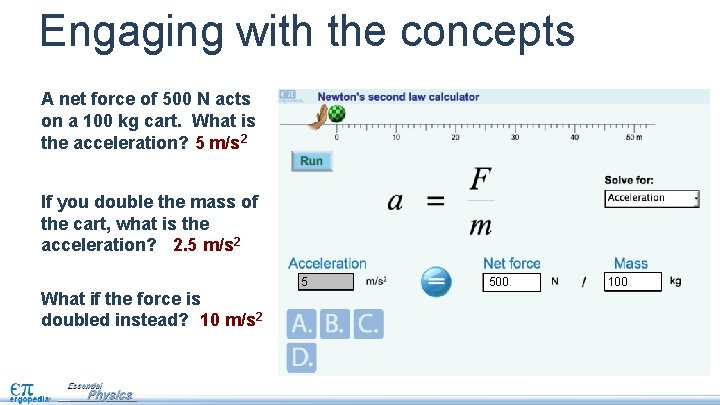Engaging with the concepts A net force of 500 N acts on a 100 kg cart. What is the acceleration? 5 m/s 2 If you double the mass of the cart, what is the acceleration? 2. 5 m/s 2 5 What if the force is doubled instead? 10 m/s 2 500 100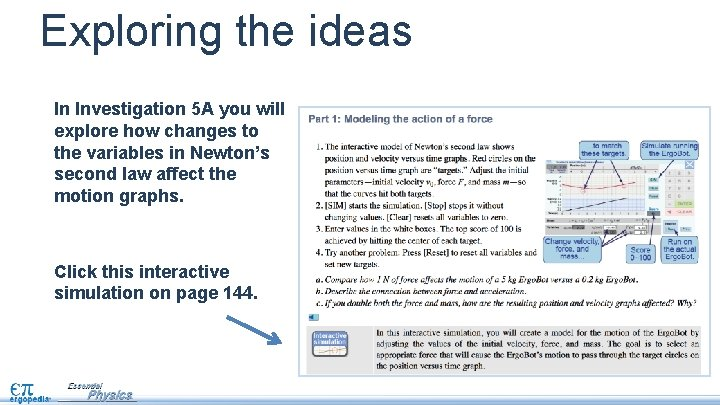Exploring the ideas In Investigation 5 A you will explore how changes to the variables in Newton’s second law affect the motion graphs. Click this interactive simulation on page 144.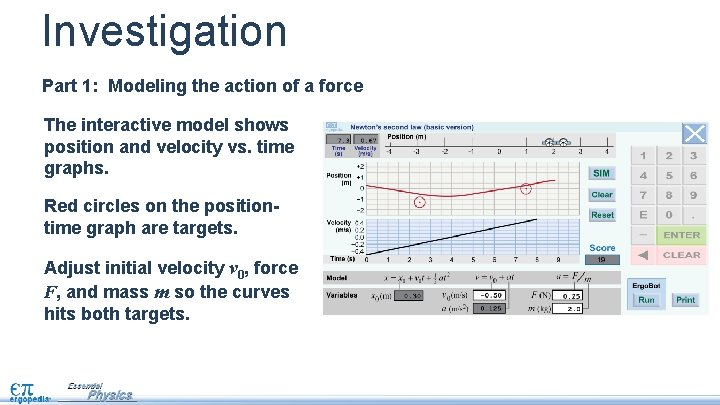Investigation Part 1: Modeling the action of a force The interactive model shows position and velocity vs. time graphs. Red circles on the positiontime graph are targets. Adjust initial velocity v 0, force F, and mass m so the curves hits both targets.Investigation Part 1: Modeling the action of a force [SIM] runs the simulation. [Stop] stops it without changing values. [Clear] resets all variables to zero. [Reset] resets all variables and sets new targets.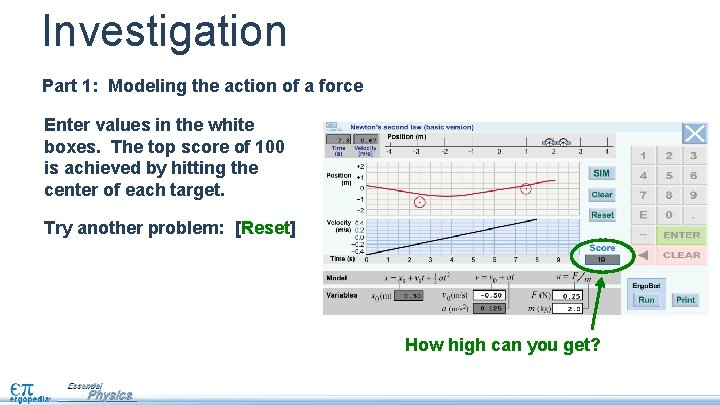Investigation Part 1: Modeling the action of a force Enter values in the white boxes. The top score of 100 is achieved by hitting the center of each target. Try another problem: [Reset] How high can you get?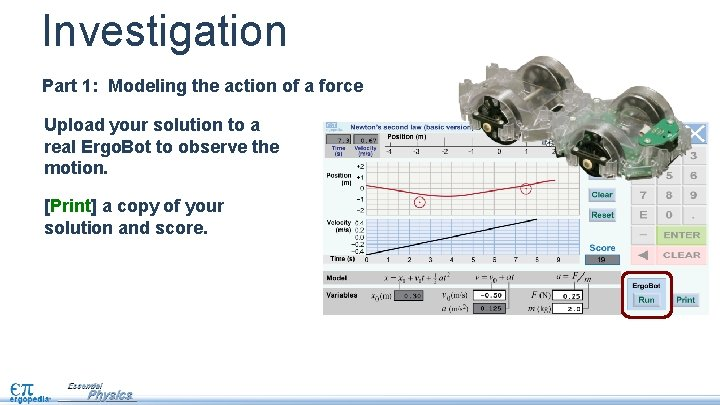Investigation Part 1: Modeling the action of a force Upload your solution to a real Ergo. Bot to observe the motion. [Print] a copy of your solution and score.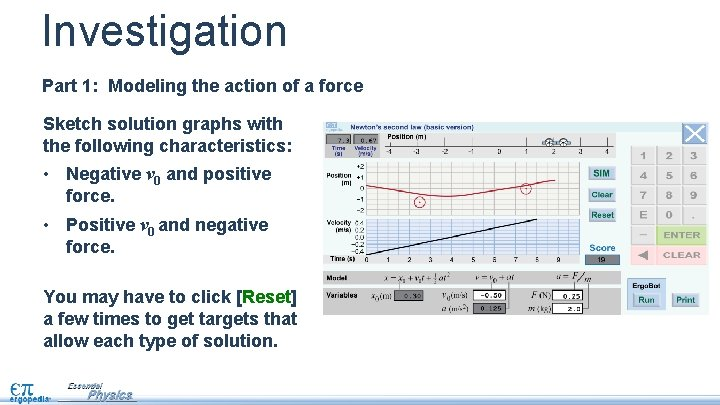Investigation Part 1: Modeling the action of a force Sketch solution graphs with the following characteristics: • Negative v 0 and positive force. • Positive v 0 and negative force. You may have to click [Reset] a few times to get targets that allow each type of solution.A tougher challenge Part 2: Dynamic modeling The second interactive simulation allows you to change the force at four different time intervals, but there also four target circles.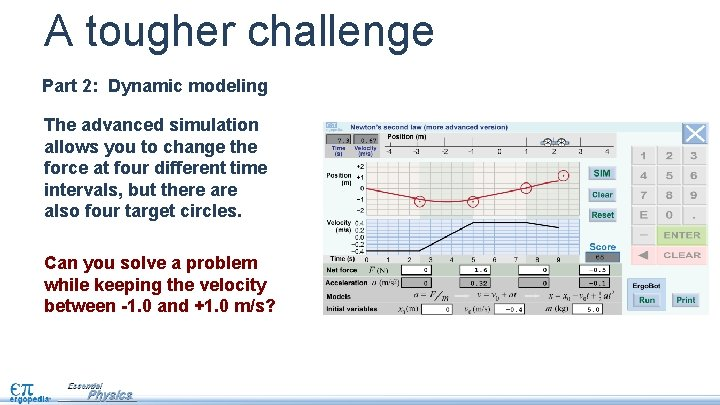A tougher challenge Part 2: Dynamic modeling The advanced simulation allows you to change the force at four different time intervals, but there also four target circles. Can you solve a problem while keeping the velocity between -1. 0 and +1. 0 m/s?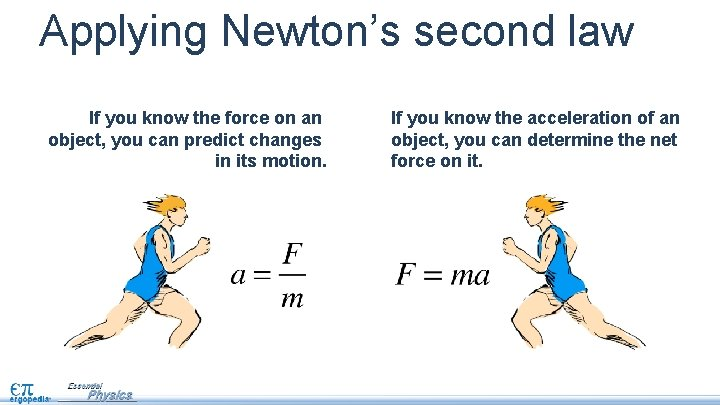Applying Newton’s second law If you know the force on an object, you can predict changes in its motion. If you know the acceleration of an object, you can determine the net force on it.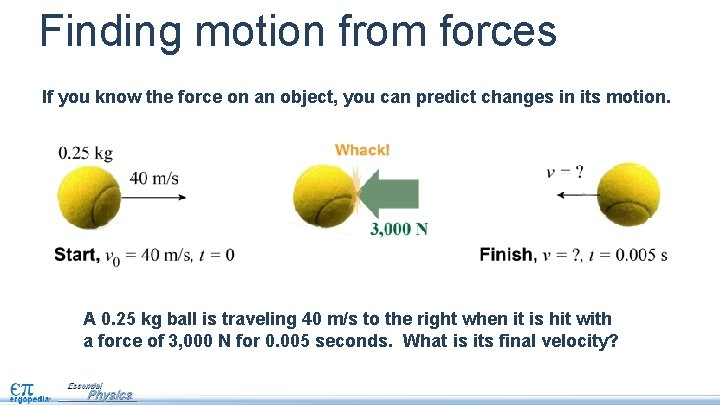Finding motion from forces If you know the force on an object, you can predict changes in its motion. A 0. 25 kg ball is traveling 40 m/s to the right when it is hit with a force of 3, 000 N for 0. 005 seconds. What is its final velocity?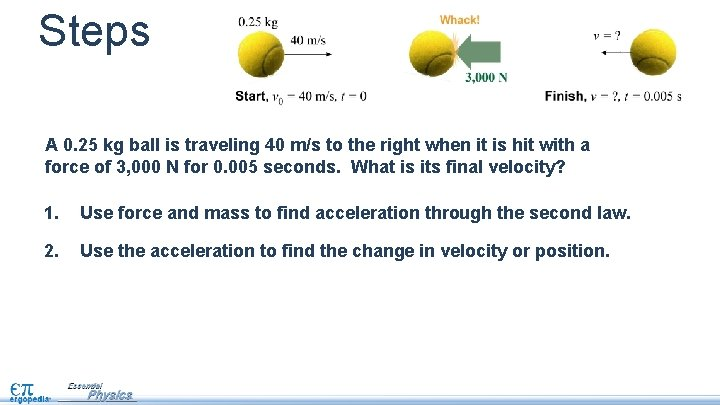Steps A 0. 25 kg ball is traveling 40 m/s to the right when it is hit with a force of 3, 000 N for 0. 005 seconds. What is its final velocity? 1. Use force and mass to find acceleration through the second law. 2. Use the acceleration to find the change in velocity or position.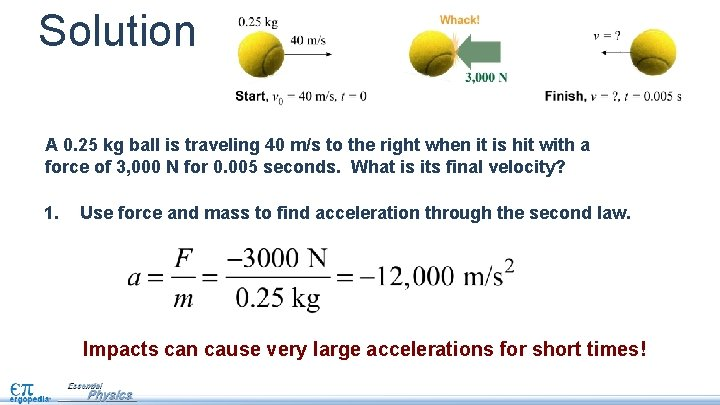Solution A 0. 25 kg ball is traveling 40 m/s to the right when it is hit with a force of 3, 000 N for 0. 005 seconds. What is its final velocity? 1. Use force and mass to find acceleration through the second law. Impacts can cause very large accelerations for short times!Solution A 0. 25 kg ball is traveling 40 m/s to the right when it is hit with a force of 3, 000 N for 0. 005 seconds. What is its final velocity? 1. Use force and mass to find acceleration through the second law. 2. Use the acceleration to find the change in velocity or position. The ball reverses direction!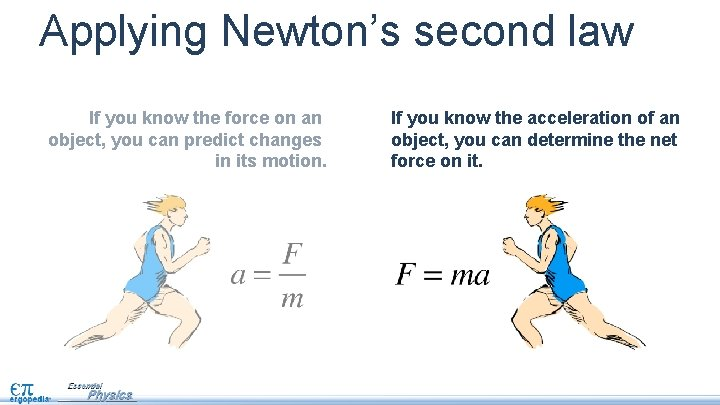Applying Newton’s second law If you know the force on an object, you can predict changes in its motion. If you know the acceleration of an object, you can determine the net force on it.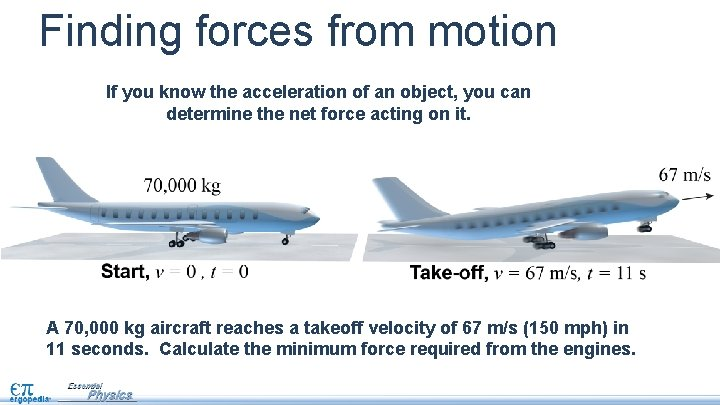Finding forces from motion If you know the acceleration of an object, you can determine the net force acting on it. A 70, 000 kg aircraft reaches a takeoff velocity of 67 m/s (150 mph) in 11 seconds. Calculate the minimum force required from the engines.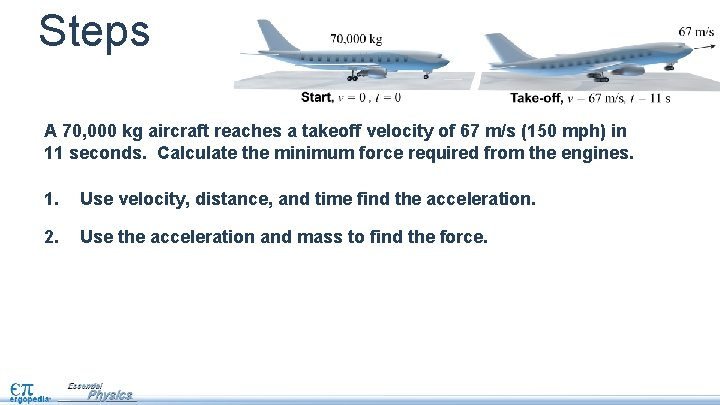Steps A 70, 000 kg aircraft reaches a takeoff velocity of 67 m/s (150 mph) in 11 seconds. Calculate the minimum force required from the engines. 1. Use velocity, distance, and time find the acceleration. 2. Use the acceleration and mass to find the force.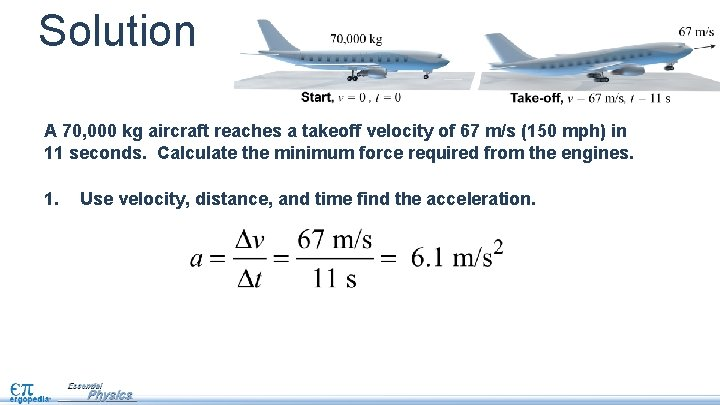Solution A 70, 000 kg aircraft reaches a takeoff velocity of 67 m/s (150 mph) in 11 seconds. Calculate the minimum force required from the engines. 1. Use velocity, distance, and time find the acceleration.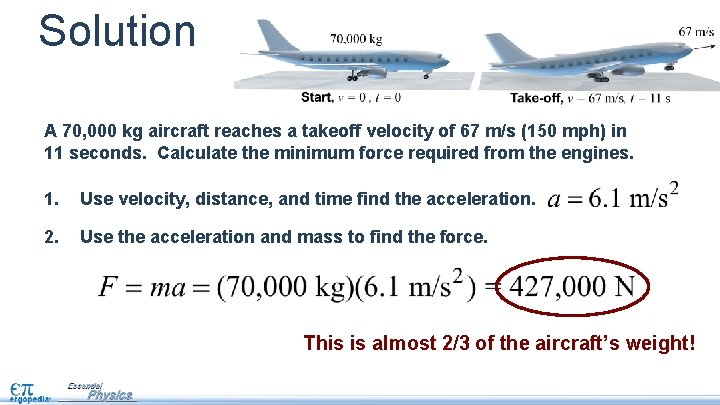Solution A 70, 000 kg aircraft reaches a takeoff velocity of 67 m/s (150 mph) in 11 seconds. Calculate the minimum force required from the engines. 1. Use velocity, distance, and time find the acceleration. 2. Use the acceleration and mass to find the force. This is almost 2/3 of the aircraft’s weight!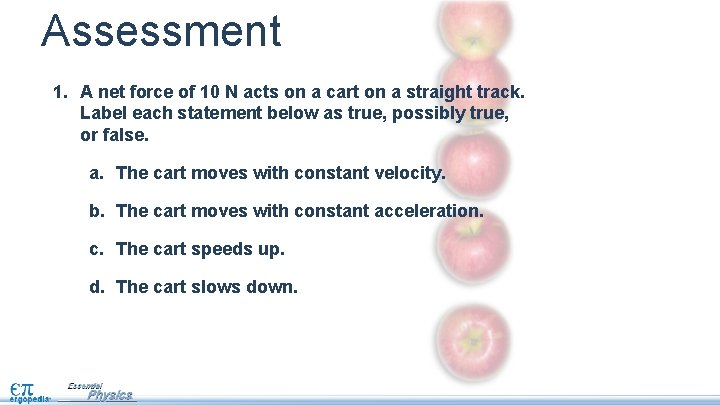Assessment 1. A net force of 10 N acts on a cart on a straight track. Label each statement below as true, possibly true, or false. a. The cart moves with constant velocity. b. The cart moves with constant acceleration. c. The cart speeds up. d. The cart slows down.Assessment 1. A net force of 10 N acts on a cart on a straight track. Label each statement below as true, possibly true, or false. a. The cart moves with constant velocity. false b. The cart moves with constant acceleration. true c. The cart speeds up. possibly true d. The cart slows down. possibly true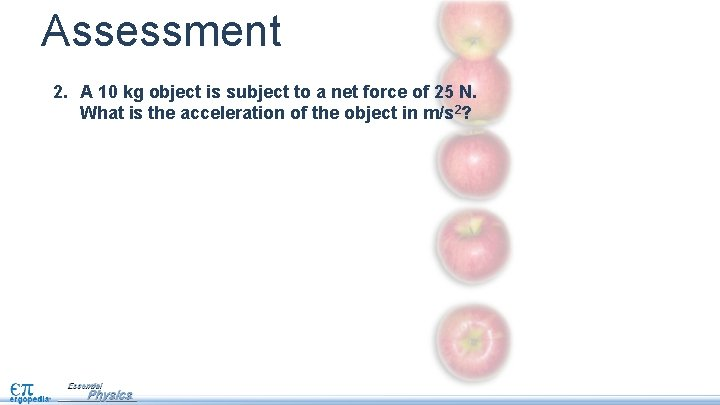Assessment 2. A 10 kg object is subject to a net force of 25 N. What is the acceleration of the object in m/s 2?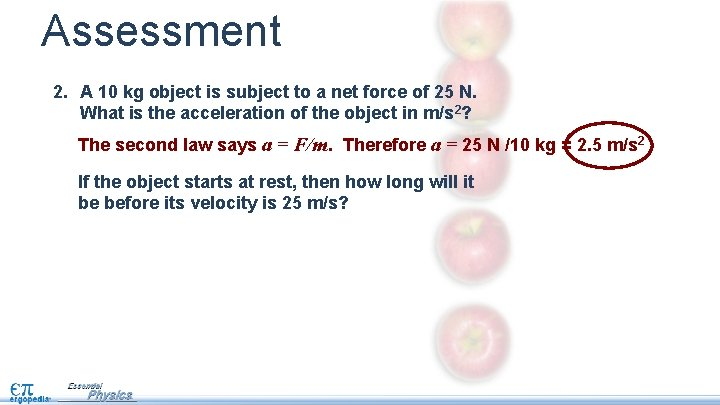Assessment 2. A 10 kg object is subject to a net force of 25 N. What is the acceleration of the object in m/s 2? The second law says a = F/m. Therefore a = 25 N /10 kg = 2. 5 m/s 2 If the object starts at rest, then how long will it be before its velocity is 25 m/s?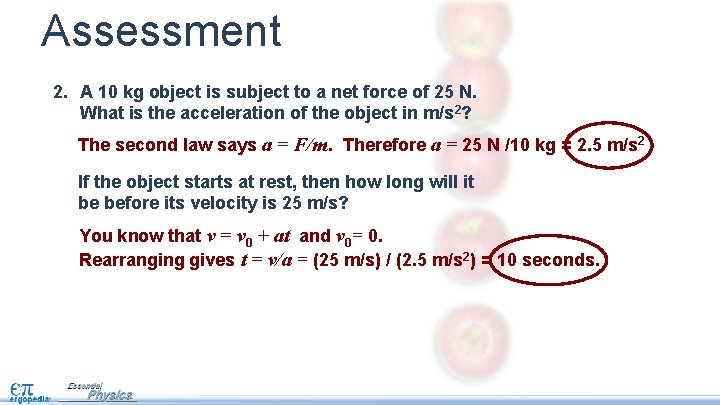Assessment 2. A 10 kg object is subject to a net force of 25 N. What is the acceleration of the object in m/s 2? The second law says a = F/m. Therefore a = 25 N /10 kg = 2. 5 m/s 2 If the object starts at rest, then how long will it be before its velocity is 25 m/s? You know that v = v 0 + at and v 0= 0. Rearranging gives t = v/a = (25 m/s) / (2. 5 m/s 2) = 10 seconds.Assessment 3. What is the minimum force required to increase the speed of a 1, 000 kg vehicle by 10 m/s in 3 seconds?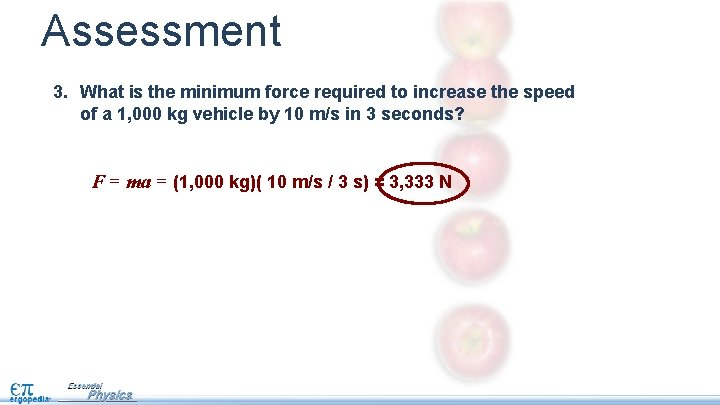Assessment 3. What is the minimum force required to increase the speed of a 1, 000 kg vehicle by 10 m/s in 3 seconds? F = ma = (1, 000 kg)( 10 m/s / 3 s) = 3, 333 N